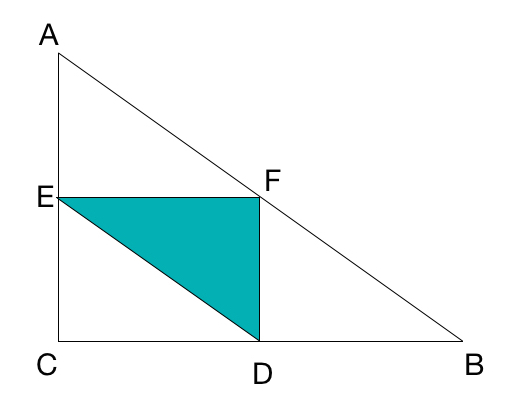# Area Of The Medial Triangle

Geometry Level 1Let $D,E,F$ be the midpoints of the sides of $\triangle ABC$.

The area of $\triangle ABC$ is $X$ and the area of $\triangle DEF$ is $Y$.

Enter your answer as $\dfrac XY$.

×

Problem Loading...

Note Loading...

Set Loading...